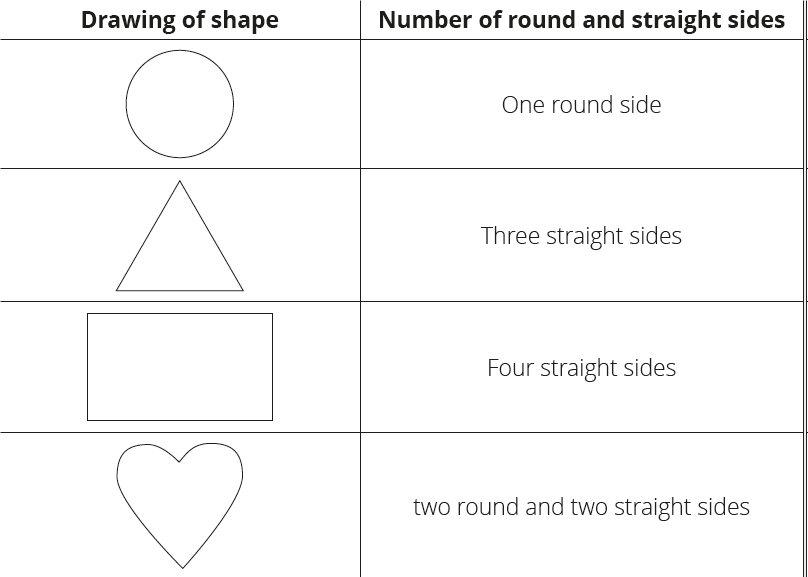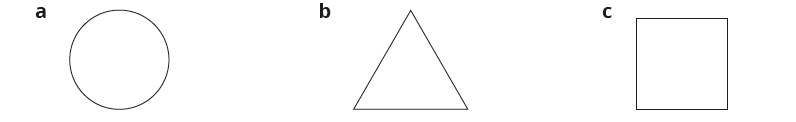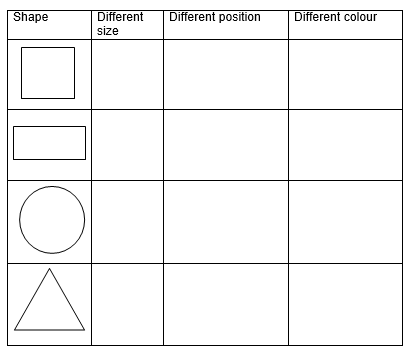# Lesson Notes By Weeks and Term - Primary 3

2-D Shapes

TERM: 2nd Term

WEEK: 6

CLASS: Primary 3

AGE: 8 years

DURATION: 5 periods of 40 minutes each

DATE:

SUBJECT: Mathematics

TOPIC: 2-D shapes

SPECIFIC OBJECTIVES: At the end of the lesson, the pupils should be able to

1. Describe and compare rectangles.
2. Sort and compare 2-D shapes.
3. Describe, sort and compare squares.

INSTRUCTIONAL TECHNIQUES: Explanation, question and answer, demonstration, practical, assessments

INSTRUCTIONAL MATERIALS: String and sticks. scrap paper, Shape cut outs (1) and shape names, scrap paper, coloured pencils, videos from source-

PERIOD 1: Rectangles

PERIOD 2 : Assessments

 PRESENTATION TEACHER’S ACTIVITY PUPIL’S ACTIVITY STEP 1ORALASSESSMENTS The teacher asks questions on 2-D shapes Pupils respond and participate STEP 2DISCUSSION The teacher discusses all the methods used by some learners in the oral assessments(some of the questions are solved on the board by the learners) and addresses any misconceptions that may have risen Pupils pay attention and participate STEP 3WRITTEN ASSESSMENTS 1 Circle which of these shapes have round sides.Squares, circle, rectangle, triangle 2 Draw 3 different size triangles in 3 different positions.3 Draw a square that has 5 cm sides.4 Draw a rectangle that is 6 cm long and 4 cm wide.5 A square, triangle, circle and rectangle are 2-D shapes. What does this mean? Pupils attempt their class work STEP 4SUMMARY The teacher marks the written assessments, corrects were necessary and commends the pupils

PERIOD 3: Sort and compare 2-D shapes

PRESENTATION

TEACHER’S ACTIVITY

PUPIL’S ACTIVITY

STEP 1

MENTAL MATHS

The teacher begins the lesson with some mental calculations

Calculate

1 1 × 1 =

2 4 × 5 =

3 5 × 2 =

4 3 × 1 =

5 7 × 3 =

6 3 × 4 =

7 2 × 1 =

8 8 × 0 =

9 9 × 10 =

10 6 × 5 =

Pupils respond and participate

STEP 2

CONCEPT

DEVELOPMENT

The teacher

Uses Prestik to stick cut-outs of the following shapes on the board: rectangle, triangle,

circle, square.

Gets the learners to identify the labels that belong to the cut-outs of the shapes and place

them below the correct shapes.

Points to one shape e.g. the rectangle, and ask the learners to each contribute one

sentence towards describing the shape, e.g. It has 4 sides/All the sides are straight/There

are 4 corners/All the corners are the same size, etc.

Does the same for the remaining shapes.

Tries to ensure that everyone gets a chance to say something using the correct vocabulary.

CLASS ACTIVITY

The teacher

Gives each group a sheet of scrap paper to draw on.

Asks learners to draw as many shapes as they can think of with straight sides on

the paper.

Every learner in the group should get a chance to draw a shape.

Compares each group’s shapes to see if there are any other shapes that they didn’t think

Gives each group a turn to call out one shape and a colour. The whole class now colours

that shape (e.g. triangles – red). If a group does not have the shape, they draw it in.

Carries on until all the shapes are coloured in.

ACTIVITY II

Revises by asking: If a shape does not have straight sides, what will it have?

(round sides)

Draws the following shapes on the board, and ask learners to identify the number of straight and round sides on each one.Pupils pay attention and participate

STEP 3

CLASS-WORK

1 Say if the following shapes have round or straight sides:2 Draw three shapes in each block below:

 Shapes with straight sides Shapes with round sides Shapes with straight and round sides

Pupils attempt their class work

STEP 4

HOME-WORK

Draw a picture of a house. Use circles, squares, rectangles and triangles.

Pupils attempt their class work

STEP 5

SUMMARY

The tacher summarizes by reminding the pupils how to sort and compare 2-D shapes.

She marks their class works, makes corrections where necessary and commends them positively

PERIOD 4: Consolidation of 2-D shapes

 PRESENTATION TEACHER’S ACTIVITY PUPIL’S ACTIVITY STEP 1MENTAL MATHS The teacher begins the lesson with some mental calculationsCalculate1 __ × 10 = 50   2 __ × 10 = 30   3 __ × 2 = 12     4 __ × 2 = 20   5 __ × 10 = 60  6 __ × 10 = 907 __ × 2 = 188 __ × 2 = 149 __ × 10 = 7010 __ × 2 = 0 Pupils respond and participate STEP 2CONCEPTDEVELOPMENT The teacher• Revises curved and straight sides by asking learners to draw different 2-D shapes onthe board.Each time, ask the learner to name the shape they drew and discuss is properties(straight/round sides, big/small).• Uses the shape name cards to label the drawings the learners do. CLASS ACTIVITYThe teacher* Sticks cardboard cut-out shapes (rectangles, squares and triangles) randomly on the board.• Asks: What can you tell me about the sides of all these shapes? (They are all straight.)• Asks: What shapes are they? (Triangles, rectangles, squares.)• Calls a learner to sort the shapes on the board.• Asks: How did you sort the shapes (We put all the rectangles together, all the triangles together and all the, squares together.)Rotates the triangles so that they all face different directions.• Asks: Are the shapes in the circle still triangles? (Yes.)• Asks: How do you know? (Triangles have three straight sides and three corners. If yourotate a shape it changes position but the shape itself does not change.) Do the same with the rectangles and squares. ACTIVITY IIThe teacher- Draws the following on the board. Ask learners to come to the board and draw other shapes,e.g. triangles that look different from yours/are facing in a different direction. Ask them toexplain what the difference is.Note: The teacher helps learners to become familiar with the shape terminology by asking questionsand allowing learners to use the words they have learnt. Pupils pay attention and participate STEP 3CLASS-WORK Learners need to use old newspapers/magazines/advertisement flyers for question 7of the classwork activity. (Answers will vary)1 Draw a triangle. Draw three more triangles, but in different positions.2 Are the sides of the triangle round or straight?3 Draw a rectangle. Draw three more rectangles, but in different positions.4 Are the sides of the rectangle round or straight?5 Draw three circles of different size.6 Are the sides of the circle round or straight?7 Find and cut out squares of different sizes from a magazine. Stick them in your book, allin different positions.a How many sides does each one have?b Are they straight or round? Pupils attempt their class work STEP 4HOME-WORK Draw a picture of a tree. You may use shapes with straight sides and round sides. Pupils attempt their class work STEP 5SUMMARY The teacher revises the properties of 2-D shapes. She marks their class works, makes corrections where necessary and commends them positively

PERIOD 5: Weekly Test/consolidations

TEACHER’S ACTIVITY: The teacher revises all the concepts treated from period 1-4 and gives the pupils follow through exercises, quiz and tests . She marks the exercises, makes corrections and commends the pupils positively.

PUPIL’S ACTIVITY: The pupils work on the worksheets and exercises given by the teacher individually

CONSOLIDATION

1 Complete the table: